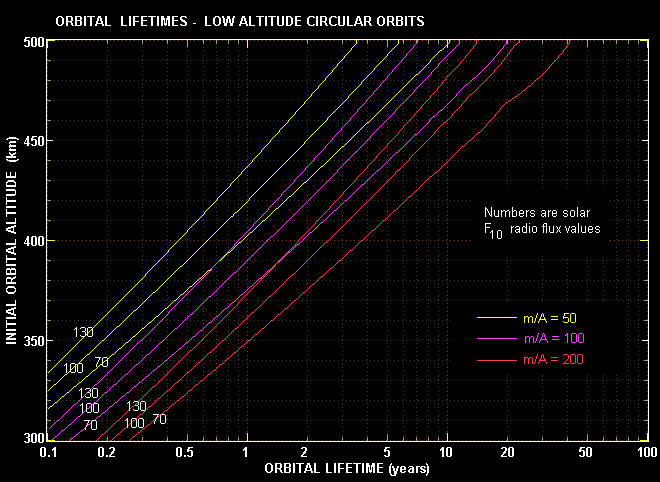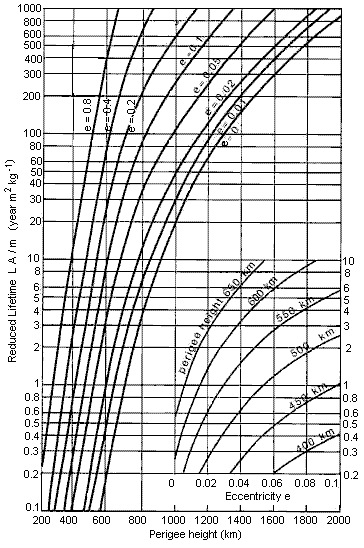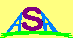INTRODUCTION

All satellites in Earth orbit are subject to various perturbing influences which can alter their orbit. Satellites in low Earth orbit, with perigee altitudes below 2000 km, are predominantly subject to atmospheric drag. This force very slowly tends to circularise and reduce the altitude of the orbit. The rate of 'decay' of the orbit becomes very rapid at altitudes less than 200 km, and by the time the satellite is down to 180 km it will only have a few hours to live before it makes a fiery re-entry down to the Earth. The temperatures attained during this re-entry are usually great enough to vapourise most of the satellite, but if it is particularly large, or under certain conditions, component pieces may reach the ground.

The rate at which a low satellite orbit decays is a function of atmospheric density at each point along the orbit together with a satellite's effective cross sectional area A, mass m, and drag coefficient CD. In many cases, these last three parameters cannot be independently determined and a ballistic coefficient [ B = CD A/m ] is used instead. This has the units of metres squared per kilogram.

The average m/A for an orbital object is around 100 kg m-2 with most objects lying between 50 and 200 kg m-2. This equates to A/m values between 0.005 and 0.02, with an average value of 0.01 m2 kg-1.The air density varies along the orbit, being a function of latitude and longitude, time of day, time of year and season. However, at a fixed point in space, if we average the short term variations, we find that the density can be expressed in terms of two space environmental parameters. These are the solar 10 cm radio flux (F10) and the geomagnetic index Ap. As each of these increase we find a corresponding increase in the atmospheric density at altitudes above about 120 km.

Over long time intervals the geomagnetic index Ap has an average value that is quite low, and is of no significance to lifetime predictions. It is only over timescales of a day or two that high geomagnetic activity can cause a significant increase in satellite drag, and then only for altitudes below about 400 km.

The graph below illustrates the typical variation in the solar radio flux (F10) at a wavelength near 10 cm. It has a strong 11 year component and a smaller periodicity around 27 days that is only pronounced at times of the 11 year cycle minimum.The uncertainty in prediction of the space environment coupled with unresolved variations in atmospheric density preclude us from being able to specify exactly when a satellite will re-enter the atmosphere. Even quite sophisticated programs can only claim a prediction accuracy not better than 10%. This means that one day before a particular re-entry is due, the uncertainty in time of fall will be at least 2 hours. The satellite will have circled the globe within this time span.

A ROUGH GUIDE

The following table provides a very rough guide to the lifetime of an object in a circular or near circular orbit at various altitudes.

 Satellite Altitude Lifetime 200 km 1 day 300 km 1 month 400 km 1 year 500 km 10 years 700 km 100 years 900 km 1000 years

The following graphs provide more detailed estimates of space object orbital lifetimes under various conditions.

VERY LOW CIRCULAR ORBITS

The graph below gives a very rough guide to the lifetime of a satellite with effective mass to cross-section ratio of 100 kg/m2 in a circular orbit below 300 km. Two cases of constant solar activity are considered, one representing solar minimum conditions, and the other solar maximum. The geomagnetic field is assumed to be quiet during this periods.This graph can be linearly scaled for other values of (m/A). For instance the graph shows a lifetime of 20 days for an initial orbit at 260 km in solar flux minimum conditions (F10 = 70). If a spacecraft had a value of m/A = 200 the lifetime would be 40 days, and a value of m/A = 50 would produce a lifetime of 10 days.

Note that the graph for maximum solar flux conditions is a little misleading as a flux of this value normally only occurs for a very few days.

LOW CIRCULAR ORBITS

The following graph displays orbital lifetimes for 3 values of solar radio flux and 3 values of m/A.ALL ORBITSThis graph is from King-Hele (1987) and displays a normalised or reduced orbital lifetime (L*) for a wide range of perigee heights and eccentricities. The actual lifetime in years can be calculated from: L = L* ( m / A ) where (m/A) is the actual mass to area ratio (kg m-2) of the object in question. Thus, if m/A = 100, the scale is multiplied by 100 to give the actual lifetime in years. The atmospheric densities used in computing these curves was taken from a model (CIRA-1972) with an exospheric temperature of 900 K, which King-Hele states is "approximately the average over a solar cycle". The inset graph gives greater detail for the lower altitude regime.

REFERENCES

Desmond King-Hele, Satellite Orbits in an Atmosphere - Theory and Applications, Blackie [Glasgow, 1987]Australian Space Academy